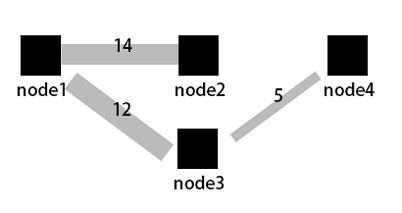Sankey diagram,  a specific type of flow chart, is used to describe the flow from one set of values to another,  and is applicable to scenarios such as network flow data. Generally, the Sankey diagram contains three sets of values: source , target , and value . source and target describes the edge relationship between nodes, and value describes the relationship between source and target .

Function features

The Sankey diagram has the following features:

• The start flow is the same as the end flow. The overall width of all the main branches is the same as that of all the branches. Therefore, the energy balance is maintained.
• Internally, different lines indicate the distribution of different flows, and the line width indicates the flow occupied by the branch proportionally.
• Different node widths indicate the flows in the particular statuses.
For example, the following data can be displayed in a Sankey diagram.
Source Target Value
node1 node2 14
node1 node3 12
node3 node4 5
..

The Sankey diagram visualizes the preceding data as follows.Figure 1. Sankey diagram data relation

• Node
• Edge

Configuration items

Configuration item Description
Start column Describes the start node.
End column Describes the end node.
A value column The value that links the start node and the end node.
Spacing The distance between the coordinate axis and the graph boundary.

Procedure

1. On the query page, enter the query statement in the search box, select the time interval, and click Search.
2. Click the Graph and select the Sankey diagram.
3. Configure the graph properties.

Ordinary Sankey diagram

If the log field contains sourcetarget, and value, each log itself is the relationship between nodes and edges, and the sum of steamValue can be obtained using Nested subquery Nested subquery.
* | select sourceValue, targetValue, sum(streamValue) as streamValue from (select sourceValue, targetValue,
streamValue, __time__ from log group by sourceValue, targetValue, streamValue, __time__ order by __time__ desc) group by sourceValue,
targetValue